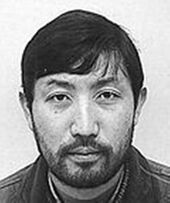# Alumnae and Alumni

 Ken-ichi Yoshikawa PositionVisiting Senior Scientist (from 2011/04/01 to 2019/03/31) Scientist　 (from 2008/02/01 to 2010/03/31) Current AffiliationDivision of Mathematics and Mathematical Analysis, Graduate School of Science, Kyoto University ProfessorLast Update 2018/08/08

My current research subject is holomorphic torsion invariant of algebraic manifolds with trivial canonical line bundle. One of the typical examples of such invariants is the one for K3 surfaces with involution, which I introduced in 2004. There are 75 deformation types of K3 surfaces with involution, and correspondingly we have 75 moduli spaces. On each moduli space, the invariant is given by the Petersson norm of a Borcherds product and its explicit formula is known. It is an interesting open problem of understanding the geometric meaning of the corresponding elliptic modular form. Another typical example is the so called BCOV invariant for Calabi-Yau threefolds, which was introduced in 1994 by Bershadsky-Cecotti-Ooguri-Vafa as the counterpart in B-model of genus-one instanton numbers of Calabi-Yau threefolds. This invariant is very important because the mirror symmetry at genus-one is formulated as the equality of two functions on the moduli spaces, where one is obtained as the generating function of genus-one instanton numbers and the other is obtained as the BCOV invariant. I computed the BCOV invariant in some classical examples (mirror quintics, Borcea-Voisin threefolds). Recently I extend the construction of BCOV invariants to abelian Calabi-Yau orbifolds of dimension three. Besides mirror symmetry at genus-one, I am interested in the problem such as the birational invariance of BCOV invariants and the comparison of BCOV invariants between Calabi-Yau orbifolds and their crepant resolutions. Very recently, I am studying holomorphic torsion invariant of higher dimensional manifolds.

Back to Member List.